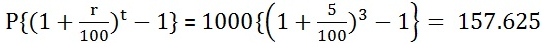## Difference between Simple Interest and Compound Interest

When we study interest chapter we usually get confused between Simple Interest and Compound Interest. So, here we will going to discuss on Difference between Simple Interest and Compound Interest because most of the students have this question in their mind while solving interest question. We will discuss difference of SI and CI in a simple and easy way with the help of Formula & Examples through which it will become easier to understand and remind it for last long.

Interest is the term that is used when a person borrows money from the money lender or any bank/financial institution then some extra amount is charged by the lending entity for the use of that money. That extra charge which is charged by lending entity is known as interest. To study in deep about Simple Interest and Compound Interest you need to go through underneath section of page that is prepared by the team members of www.privatejobshub.in. So, go ahead and learn about Simple Interest Vs Compound Interest.

### What is the Difference between Simple Interest and Compound Interest

Interest can be charged in two ways that is simple interest and compound interest. The former is the type of interest where the interest is charged only on loaned amount but in the case of the latter interest is calculated on the amount lent plus accumulated interest.

So, Simple Interest is the sum paid for using the borrowed money, for a fixed period. On the other hand, whenever the interest becomes due for payment, it is added to the principal on whom interest for the succeeding period is considered, this is known as Compound Interest.

Learn Simple Interest and Compound Interest Formula’s

 Formula: Simple Interest = P×I×N

Where,
• P = Principal Amount
• I = rate of interest
• N = number of years
 Formula: Compound Interest = P {(1 + i) n – 1}

Where,
• P = Principal
• N = number of years
• I = rate of interest per period

Difference between Simple Interest and Compound Interest

 BASIS FOR COMPARISON SIMPLE INTEREST COMPOUND INTEREST Meaning Simple Interest refers to an interest that is calculated as a percentage of the principal amount. Compound Interest refers to an interest which is calculated as a percentage of principal and accrued interest. Interest charged on Principal Principal + Accumulated Interest Principal Constant Goes on changing during the entire borrowing period. Return Less Comparatively high Growth Remains uniform Increases rapidly Formula Simple Interest = P*r*n Compound Interest = P*(1 + r)^nk

Example for CI and SI

Question: Suppose Alex deposited Rs. 1000 to a bank at 5% interest (simple and compound) p.a. for 3 years. Find out the total interest that he will get at the end of the third year?

Solution: Here P = 1000, r = 5% and t = 3 years

Simple interest =Compound interest =Conclusion

By going through above information, it is understood that Interest is the fee for using someone else’s money. There are many reasons for paying interest like time value of money, inflation, opportunity cost, and risk factor.

Simple Interest is quick to calculate on the other hand Compound Interest is practically difficult. If you compute, both simple interest and compound interest for a given Principal, Rate, and Time, you will always find that compound interest is always higher than the simple interest due to the compounding effect on it. This you can judge by going through above example.

 Check Some Other Important Maths Preparation Links

Final Note:

You can go through the prepared page to know about Difference between Simple Interest and Compound Interest with the help of Formula & Examples. If you face any query or any suggestion related to this you can leave a comment in below mentioned comment box our team will soon reply towards it. Also, bookmark our web page that is www.privatejobshub.in to get latest and instant updates.